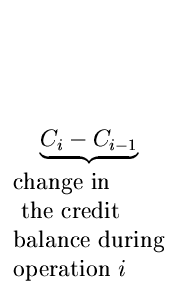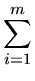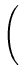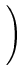Next:4.6.4 Example of Incrementing Binary IntegersUp:4.6 Amortized Algorithm AnalysisPrevious:4.6.2 Example of Tree Traversal (Inorder)

## 4.6.3 Credit Balance

• The credit function behaves like the bank balance of a well-budgeted family. It will be large when the next operation is expensive and smaller when the next operation can be done quickly.
• Consider a sequence of m operations on a data structure. Let
ti = Actual cost of operation i for    1im
Let the values of the credit between balance function be

C0, C1, C2,..., Cm

 C0 = Credit balance before the first operation Ci = Credit balance after operation  i

Amortized Cost
• The amortized cost ai of each operation i is defined by
ai = tichoose the credit balance function Ci so as to make the amortized costs ai as nearly equal as possible, no matter how the actual costs may vary.

We have

 ti = ai - Ci + Ci - 1 Henceti = (a1 - C1 + C0) + (a2 - C2 + C1) + ... + (am - Cm + Cm - 1) =ai+ (C0 - Cm)

Thustiai+ C0 - Cm
• Thus the total actual cost can be computed in terms of the amortized costs and the initial and final values of the credit balance function.Next:4.6.4 Example of Incrementing Binary IntegersUp:4.6 Amortized Algorithm AnalysisPrevious:4.6.2 Example of Tree Traversal (Inorder)
eEL,CSA_Dept,IISc,Bangalore